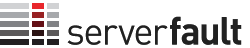0

(click on this box to dismiss)Q&A for system and network administrators

SELECT
COUNT(p.id) as posts,
CAST(
CAST(COUNT(v.id) as DECIMAL(20,5))
/
CAST(COUNT(p.id) as DECIMAL(20,5))
as DECIMAL(20,5)) as voteweightUp
left join posts p
on p.id = v.postid
where v.VoteTypeId in ('2')
and v.creationdate > '2014-01-01'

UNION ALL

SELECT
COUNT(p.id) as posts,
CAST(
CAST(COUNT(v.id) as DECIMAL(20,5))
/
CAST(COUNT(p.id) as DECIMAL(20,5))
as DECIMAL(20,5)) as voteweightHold tight while we fetch your results